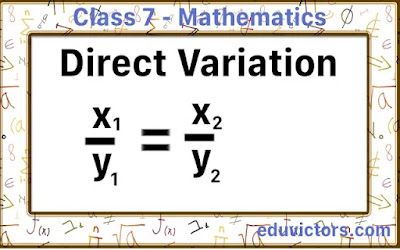CBSE Class 7 - Maths - Solved Problems on Direct Variation

If two varying quantities are always in the same ratio, they are said to vary directlt or are directly proportional to one another. Or we simpley say that they are proportional.

In direct variation, as one quantity increases, the other quatity also increases.

If a and b are the two quantities in direct variation then, a ∝ b

e.g. At constant speed, distance covered varies directly as time, i.e., the longer the time an objec travels, the greater the distance it will cover.

This is expressed as, distance travelled ∝ time.

If $a_1$ distance takes $b_1$ time and $a_2$ distance takes $b_2$ time, then
$\frac{a_1}{b_1} = \frac{a_2}{b_2} = constant$

or we can write $a_1:a_2 = b_1:b_2$

We can solve direct variation problems by two methods:

1. Unitary method
2. By ratio method

Q1: If the cost of 6 glasses is ₹ 90, find the cost of 24 glasses.

By ratio method:

Let the cost of 24 glasses be x.

More number of glasses, more will be the cost. It is a case of direct variation.
∴ Ratio of number of glasses = Ratio of number of rupees.

∴ 6 : 24 = 90:x

∴ $\frac{6}{90} = \frac{24}{x}$

$6x = 24 \times 90$

$x = \frac{24 \times 90}{6} = 360$

∴ Cost of 24 glasses is ₹360.

Q2: If 10 persons can make 20 articles in a day, then how many persons are required to make 10 articles in a day?

Answer: Let the number of persons required be x.
The number of persons varies directly with the number of articles in a constant period.

∴ $\frac{10}{20} = \frac{x}{10}$
∴ $x = \frac{100}{20}$ = 5

Q3: Check $\frac{x}{y} = \frac{5}{15} = \frac{3}{9} = \frac{11}{33}$ are in direct variation or not?

Answer: Since $\frac{x}{y} = \frac{5}{15} = \frac{3}{9} = \frac{11}{33} = \frac{1}{3}$ is constant.
∴ x and y are in direct variation.

Q4: The quantities x and y are in direct variation. Find the missing values x₁, x₂ and y₁.
$\frac{x}{y} = \frac{3}{72} = \frac{x_1}{120} = \frac{x_2}{192} = \frac{10}{y_1}$

$\frac{x}{y} = \frac{3}{72} = \frac{x_1}{120} = \frac{x_2}{192} = \frac{10}{y_1}$

∴ $\frac{3}{72} = \frac{x_1}{120}$
∴ $\Rightarrow x_1 = \frac{120\times3}{72} = 5$

$\frac{3}{72} = \frac{x_2}{192}$
∴ $\Rightarrow x_2 = \frac{192\times3}{72} = 8$

$\frac{3}{72}= \frac{10}{y_1}$
∴ $\Rightarrow y_1 = \frac{72\times10}{3} = 240$